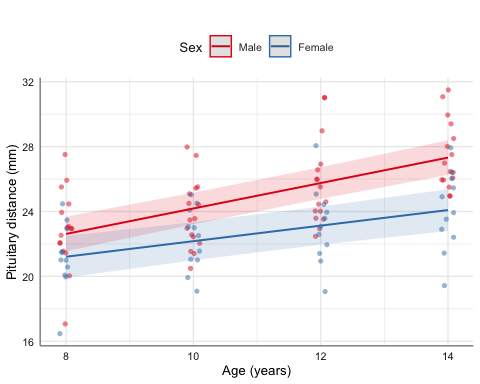# 1 Introduction

The aim of this vignette is to illustrate the use/functionality of the glm_coef function. glm_coef can be used to display model coefficients with confidence intervals and p-values. The advantages and limitations of glm_coef are:

1. Recognises the main models used in epidemiology/public health.
2. Automatically back-transforms estimates and confidence intervals, when the model requires it.
3. Can use robust standard errors for the calculation of confidence intervals.
• Standard errors are used by default.
• The use of standard errors is restricted by the following classes of objects (models): gee, glm and survreg.
4. Can display nice labels for the names of the parameters.
5. Returns a data frame that can be modified and/or exported as tables for publications (with further editing).

We start by loading relevant packages and setting the theme for the plots (as suggested in the Template of this package):

rm(list = ls())
library(car)
library(broom)
library(mosaic)
library(tidyverse)
library(ggfortify)
library(huxtable)
library(jtools)
library(latex2exp)
library(pubh)
library(sjlabelled)
library(sjPlot)
library(sjmisc)

theme_set(sjPlot::theme_sjplot2(base_size = 10))
theme_update(legend.position = "top")
options('huxtable.knit_print_df' = FALSE)
options('huxtable.autoformat_number_format' = list(numeric = "%5.2f"))
knitr::opts_chunkset(comment = NA) # 2 Multiple Linear Regression For continuous outcomes there is no need of exponentiating the results unless the outcome was fitted in the log-scale. In our first example we want to estimate the effect of smoking and race on the birth weight of babies. We can generate factors and assign labels in the same pipe stream: data(birthwt, package = "MASS") birthwt <- birthwt %>% mutate( smoke = factor(smoke, labels = c("Non-smoker", "Smoker")), race = factor(race, labels = c("White", "African American", "Other")) ) %>% var_labels( bwt = 'Birth weight (g)', smoke = 'Smoking status', race = 'Race' ) Is good to start with some basic descriptive statistics, so we can compare the birth weight between groups. birthwt %>% group_by(race, smoke) %>% summarise( n = n(), Mean = mean(bwt, na.rm = TRUE), SD = sd(bwt, na.rm = TRUE), Median = median(bwt, na.rm = TRUE), CV = rel_dis(bwt) ) %>% as_hux() %>% theme_pubh(1) summarise() has grouped output by 'race'. You can override using the .groups argument. racesmokenMeanSDMedianCV WhiteNon-smoker443428.75710.103593.00 0.21 WhiteSmoker522826.85626.472775.50 0.22 African AmericanNon-smoker162854.50621.252920.00 0.22 African AmericanSmoker102504.00637.062381.00 0.25 OtherNon-smoker552815.78709.352807.00 0.25 OtherSmoker122757.17810.043146.50 0.29 Graphical analysis: birthwt %>% box_plot(bwt ~ smoke, fill = ~ race) %>% axis_labs()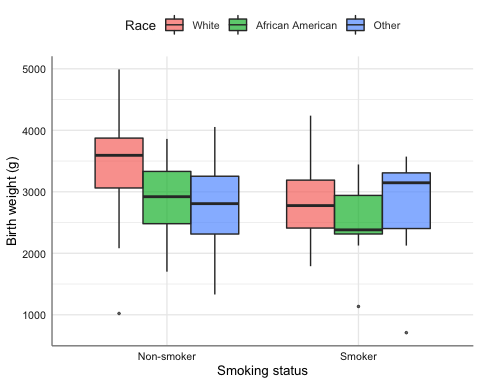Another way to compare the means between the groups is with gen_bst_df which estimates means with corresponding bootstrapped CIs. birthwt %>% gen_bst_df(bwt ~ race|smoke) %>% as_hux() %>% theme_pubh(1) Birth weight (g)LowerCIUpperCIRaceSmoking status 3428.753196.653632.94WhiteNon-smoker 2826.852663.243005.51WhiteSmoker 2854.502541.353145.55African AmericanNon-smoker 2504.002151.522826.57African AmericanSmoker 2815.782646.762980.76OtherNon-smoker 2757.172250.323151.51OtherSmoker We fit a linear model. model_norm <- lm(bwt ~ smoke + race, data = birthwt) Note: Model diagnostics are not be discussed in this vignette. Traditional output from the model: model_norm %>% Anova() Anova Table (Type II tests) Response: bwt Sum Sq Df F value Pr(>F) smoke 7322575 1 15.4588 0.0001191 *** race 8712354 2 9.1964 0.0001557 *** Residuals 87631356 185 --- Signif. codes: 0 '***' 0.001 '**' 0.01 '*' 0.05 '.' 0.1 ' ' 1 model_norm %>% summ(confint = TRUE, model.info = FALSE)  F(3,185) 8.68 R² 0.12 Adj. R² 0.11 Est. 2.5% 97.5% t val. p (Intercept) 3334.95 3153.89 3516.01 36.34 0.00 smokeSmoker -428.73 -643.86 -213.60 -3.93 0.00 raceAfrican American -450.36 -752.45 -148.27 -2.94 0.00 raceOther -452.88 -682.67 -223.08 -3.89 0.00 Standard errors: OLS Table of coefficients for publication: model_norm %>% glm_coef(labels = model_labels(model_norm)) %>% as_hux() %>% set_align(everywhere, 2:3, "right") %>% theme_pubh(1) %>% add_footnote(get_r2(model_norm), font_size = 9) ParameterCoefficientPr(>|t|) Constant3334.95 (3153.89, 3516.01)< 0.001 Smoking status: Smoker-428.73 (-643.86, -213.6)< 0.001 Race: African American-450.36 (-752.45, -148.27)0.004 Race: Other-452.88 (-682.67, -223.08)< 0.001 R2 = 0.123 Function glm_coef allows the use of robust standard errors. model_norm %>% glm_coef(se_rob = TRUE, labels = model_labels(model_norm)) %>% as_hux() %>% set_align(everywhere, 2:3, "right") %>% theme_pubh(1) %>% add_footnote(paste( get_r2(model_norm), "\n", "CIs and p-values estimated with robust standard errors."), font_size = 9) ParameterCoefficientPr(>|t|) Constant3334.95 (3144.36, 3525.53)< 0.001 Smoking status: Smoker-428.73 (-652.88, -204.58)< 0.001 Race: African American-450.36 (-734.09, -166.63)0.002 Race: Other-452.88 (-701.4, -204.35)< 0.001 R2 = 0.123 CIs and p-values estimated with robust standard errors. The function glance from the broom package allow us to have a quick look at statistics related with the model. model_norm %>% glance() # A tibble: 1 x 12 r.squared adj.r.squared sigma statistic p.value df logLik AIC BIC <dbl> <dbl> <dbl> <dbl> <dbl> <dbl> <dbl> <dbl> <dbl> 1 0.123 0.109 688. 8.68 2.03e-5 3 -1501. 3012. 3028. # … with 3 more variables: deviance <dbl>, df.residual <int>, nobs <int> To construct the effect plot, we can use plot_model from the sjPlot package. The advantage of plot_model is that recognises labelled data and uses that information for annotating the plots. model_norm %>% plot_model("pred", terms = ~race|smoke, dot.size = 1.5, title = "")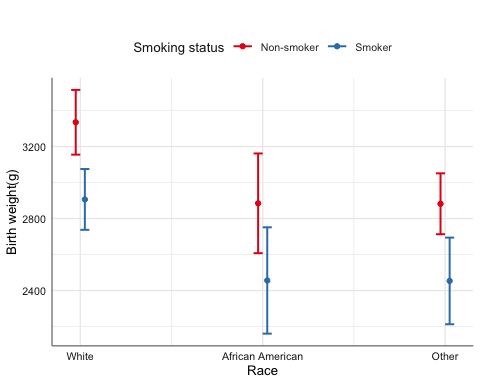When the explanatory variables are categorical, another option is emmip from the emmeans package. We can include CIs in emmip but as estimates are connected, the resulting plots look more messy, so I recommend emmip to look at the trace. emmip(model_norm, smoke ~ race) %>% gf_labs(y = get_label(birthwtbwt), x = "", col = "Smoking status")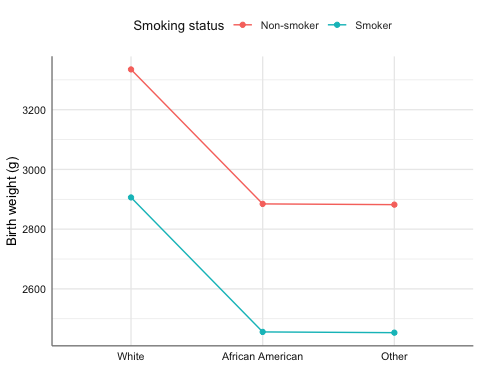# 3 Logistic Regression

For logistic regression we are interested in the odds ratios. We will look at the effect of amount of fibre intake on the development of coronary heart disease.

data(diet, package = "Epi")
diet <- diet %>%
mutate(
chd = factor(chd, labels = c("No CHD", "CHD"))
) %>%
var_labels(
chd = "Coronary Heart Disease",
fibre = "Fibre intake (10 g/day)"
)

diet %>% estat(~ fibre|chd) %>%
as_hux() %>% theme_pubh(1)
Coronary Heart DiseaseNMin.Max.MeanMedianSDCV
Fibre intake (10 g/day)No CHD288.00 0.60 5.35 1.75 1.69 0.58 0.33
CHD45.00 0.76 2.43 1.49 1.51 0.40 0.27
diet %>% na.omit() %>%
copy_labels(diet) %>%
box_plot(fibre ~ chd) %>%
axis_labs()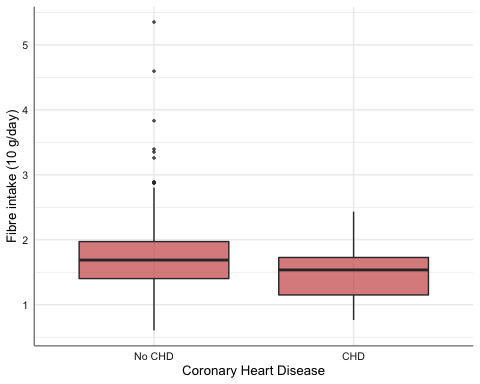We fit a linear logistic model:

model_binom <- glm(chd ~ fibre, data = diet, family = binomial)

Summary:

model_binom %>%
summ(confint = TRUE, model.info = FALSE, exp = TRUE)
 𝛘²(1) 10.22 Pseudo-R² (Cragg-Uhler) 0.06 Pseudo-R² (McFadden) 0.04 AIC 257.53 BIC 265.15
exp(Est.) 2.5% 97.5% z val. p
(Intercept) 0.95 0.29 3.11 -0.08 0.94
fibre 0.33 0.15 0.69 -2.94 0.00
Standard errors: MLE

Table of coefficients for publication:

model_binom %>%
glm_coef(labels = model_labels(model_binom)) %>%
as_hux() %>% set_align(everywhere, 2:3, "right") %>%
theme_pubh(1) %>%
add_footnote(get_r2(model_binom), font_size = 9)
ParameterOdds ratioPr(>|z|)
Constant0.95 (0.29, 3.11) 0.94
Fibre intake (10 g/day)0.33 (0.15, 0.69) 0.00
Tjur's R2 = 0.028

Effect plot:

model_binom %>%
plot_model("pred", terms = "fibre [all]", title = "")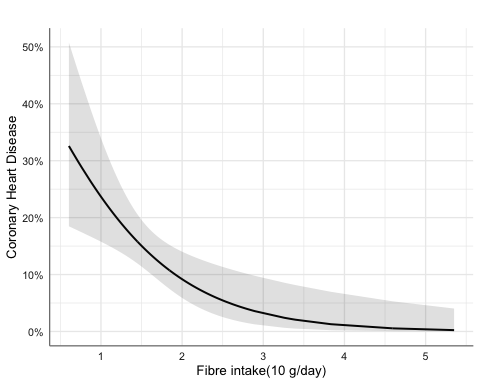## 3.1 Matched Case-Control Studies: Conditional Logistic Regression

We will look at a matched case-control study on the effect of oestrogen use and history of gall bladder disease on the development of endometrial cancer.

data(bdendo, package = "Epi")
bdendo <- bdendo %>%
mutate(
cancer = factor(d, labels = c('Control', 'Case')),
gall = factor(gall, labels = c("No GBD", "GBD")),
est = factor(est, labels = c("No oestrogen", "Oestrogen"))
) %>%
var_labels(
cancer = 'Endometrial cancer',
gall = 'Gall bladder disease',
est = 'Oestrogen'
)

t1 = bdendo %>%
filter(est == "Oestrogen") %>%
mutate(
cancer = relevel(cancer, ref = "Case"),
gall = relevel(gall, ref = "GBD")
) %>%
copy_labels(bdendo) %>%
cross_tab(cancer ~ gall,
label = "Oestrogen users")

t2 = bdendo %>%
filter(est == "No oestrogen") %>%
mutate(
cancer = relevel(cancer, ref = "Case"),
gall = relevel(gall, ref = "GBD")
) %>%
copy_labels(bdendo) %>%
cross_tab(cancer ~ gall,
label = "Non-oestrogen users")

rbind(t1, t2) %>%
theme_pubh(c(3, 6, 9))
Oestrogen users
CaseControlTotal
(N=56)(N=127)(N=183)
- GBD 13 (23.2%)16 (12.6%)29 (15.8%)
- No GBD 43 (76.8%)111 (87.4%)154 (84.2%)
Non-oestrogen users
CaseControlTotal
(N=7)(N=125)(N=132)
- GBD 4 (57.1%)8 ( 6.4%)12 ( 9.1%)
- No GBD 3 (42.9%)117 (93.6%)120 (90.9%)
bdendo %>%
gf_percents(~ cancer|gall, fill = ~ est, position = "dodge", alpha = 0.6) %>%
gf_labs(
y = "Percent",
x = "",
fill = ""
)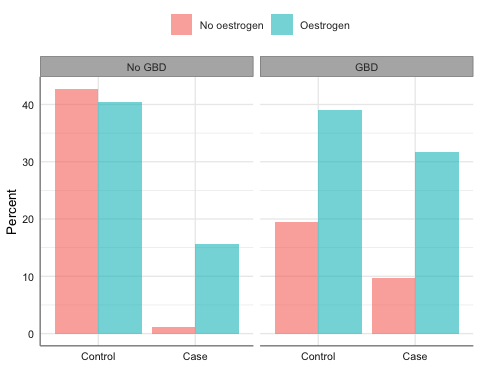We fit the conditional logistic model:

require(survival, quietly = TRUE)
model_clogit <- clogit(cancer == 'Case' ~ est * gall + strata(set), data = bdendo)

model_clogit %>%
glm_coef(labels = c("Oestrogen/No oestrogen", "GBD/No GBD",
"Oestrogen:GBD Interaction")) %>%
as_hux() %>% set_align(everywhere, 2:3, "right") %>%
theme_pubh(1) %>%
add_footnote(get_r2(model_clogit), font_size = 9)
ParameterOdds ratioPr(>|z|)
Oestrogen/No oestrogen14.88 (4.49, 49.36)< 0.001
GBD/No GBD18.07 (3.2, 102.01)0.001
Oestrogen:GBD Interaction0.13 (0.02, 0.9)0.039
Nagelkerke's R2 = 0.305

Creating data frame needed to construct the effect plot:

require(ggeffects, quietly = TRUE)
bdendo_pred <- ggemmeans(model_clogit, terms = c('gall', 'est'))

Effect plot:

bdendo_pred %>%
gf_pointrange(predicted + conf.low + conf.high ~ x|group, col = ~ x) %>%
levels(quineSex) <- c("Female", "Male") quine <- quine %>% var_labels( Days = "Number of absent days", Eth = "Ethnicity", Age = "Age group" ) Descriptive statistics: quine %>% group_by(Eth, Sex, Age) %>% summarise( n = n(), Mean = mean(Days, na.rm = TRUE), SD = sd(Days, na.rm = TRUE), Median = median(Days, na.rm = TRUE), CV = rel_dis(Days) ) %>% as_hux() %>% theme_pubh(1) EthSexAgenMeanSDMedianCV AboriginalFemaleF0517.6017.3711.00 0.99 AboriginalFemaleF11518.8716.3313.00 0.87 AboriginalFemaleF2932.5627.3120.00 0.84 AboriginalFemaleF3914.5614.8510.00 1.02 AboriginalMaleF0811.50 7.2312.00 0.63 AboriginalMaleF15 9.60 4.51 7.00 0.47 AboriginalMaleF21130.9117.8132.00 0.58 AboriginalMaleF3727.1410.3728.00 0.38 WhiteFemaleF0519.80 9.6820.00 0.49 WhiteFemaleF117 7.76 6.48 6.00 0.83 WhiteFemaleF210 5.70 4.97 4.00 0.87 WhiteFemaleF31013.5011.4912.00 0.85 WhiteMaleF0913.5620.85 7.00 1.54 WhiteMaleF19 5.56 5.39 5.00 0.97 WhiteMaleF21015.2012.8812.00 0.85 WhiteMaleF3727.2922.9327.00 0.84 quine %>% box_plot(Days ~ Age|Sex, fill = ~ Eth) %>% axis_labs() %>% gf_labs(fill = "")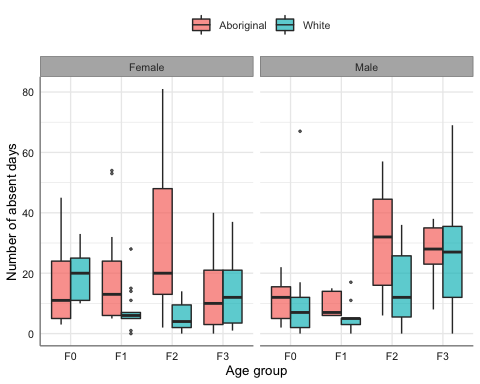We start by fitting a standard Poisson linear regression model: model_pois <- glm(Days ~ Eth + Sex + Age, family = poisson, data = quine) model_pois %>% glm_coef(labels = model_labels(model_pois), se_rob = TRUE) %>% as_hux() %>% set_align(everywhere, 2:3, "right") %>% theme_pubh(1) %>% add_footnote(get_r2(model_pois), font_size = 9) ParameterRate ratioPr(>|z|) Constant17.66 (11.08, 28.16)< 0.001 Ethnicity: White0.59 (0.43, 0.81)0.001 Sex: Male1.11 (0.81, 1.52)0.51 Age group: F10.8 (0.48, 1.32)0.38 Age group: F21.42 (0.87, 2.31)0.16 Age group: F31.35 (0.81, 2.24)0.255 Nagelkerke's R2 = 0.896 model_pois %>% glance() # A tibble: 1 x 8 null.deviance df.null logLik AIC BIC deviance df.residual nobs <dbl> <int> <dbl> <dbl> <dbl> <dbl> <int> <int> 1 2074. 145 -1165. 2343. 2361. 1743. 140 146 ## 4.1 Negative-binomial The assumption is that the mean is equal than the variance. If that is the case, deviance should be close to the degrees of freedom of the residuals (look at the above output from glance). In other words, the following calculation should be close to 1: deviance(model_pois) / df.residual(model_pois)  12.44646 Thus, we have over-dispersion. One option is to use a negative binomial distribution. model_negbin <- glm.nb(Days ~ Eth + Sex + Age, data = quine) model_negbin %>% glm_coef(labels = model_labels(model_negbin), se_rob = TRUE) %>% as_hux() %>% set_align(everywhere, 2:3, "right") %>% theme_pubh(1) %>% add_footnote(get_r2(model_negbin), font_size = 9) ParameterRate ratioPr(>|z|) Constant20.24 (12.72, 32.21)< 0.001 Ethnicity: White0.57 (0.41, 0.78)< 0.001 Sex: Male1.07 (0.78, 1.45)0.688 Age group: F10.69 (0.42, 1.16)0.16 Age group: F21.2 (0.72, 2)0.492 Age group: F31.29 (0.74, 2.23)0.369 Nagelkerke's R2 = 0.21 model_negbin %>% glance() Warning: Tidiers for objects of class negbin are not maintained by the broom team, and are only supported through the glm tidier method. Please be cautious in interpreting and reporting broom output. # A tibble: 1 x 8 null.deviance df.null logLik AIC BIC deviance df.residual nobs <dbl> <int> <dbl> <dbl> <dbl> <dbl> <int> <int> 1 192. 145 -548. 1110. 1131. 168. 140 146 Notice that age group is a factor with more than two levels and is significant: model_negbin %>% Anova() Analysis of Deviance Table (Type II tests) Response: Days LR Chisq Df Pr(>Chisq) Eth 12.6562 1 0.0003743 *** Sex 0.1486 1 0.6998722 Age 9.4844 3 0.0234980 * --- Signif. codes: 0 '***' 0.001 '**' 0.01 '*' 0.05 '.' 0.1 ' ' 1 Thus, we want to report confidence intervals and $$p$$-values adjusted for multiple comparisons. Effect plot: model_negbin %>% plot_model(type = "pred", terms = c("Age", "Eth"), dot.size = 1.5, title = "")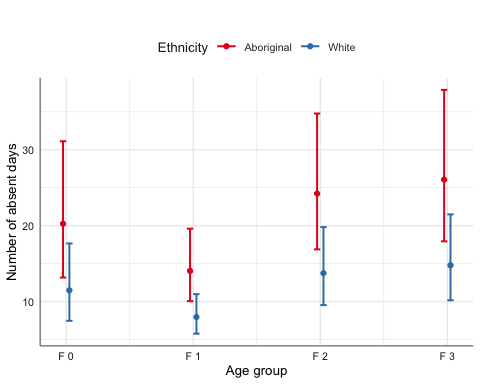emmip(model_negbin, Eth ~ Age|Sex) %>% gf_labs(y = "Number of absent days", x = "Age group", col = "Ethnicity")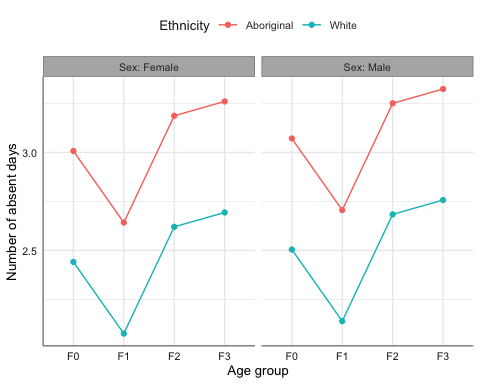## 4.2 Adjusting CIs and p-values for multiple comparisons We adjust for multiple comparisons: multiple(model_negbin, ~ Age|Eth)df %>%
as_hux() %>% theme_pubh(1)
contrastEthratioSEz.ratiop.valuelower.CLupper.CL
F1 / F0Aboriginal 0.69 0.16-1.57 0.40 0.38 1.26
F2 / F0Aboriginal 1.20 0.28 0.77 0.86 0.66 2.17
F2 / F1Aboriginal 1.73 0.35 2.65 0.04 1.02 2.92
F3 / F0Aboriginal 1.29 0.31 1.04 0.72 0.69 2.40
F3 / F1Aboriginal 1.86 0.40 2.89 0.02 1.07 3.21
F3 / F2Aboriginal 1.08 0.23 0.34 0.99 0.62 1.88
F1 / F0White 0.69 0.16-1.57 0.40 0.38 1.26
F2 / F0White 1.20 0.28 0.77 0.86 0.66 2.17
F2 / F1White 1.73 0.35 2.65 0.04 1.02 2.92
F3 / F0White 1.29 0.31 1.04 0.72 0.69 2.40
F3 / F1White 1.86 0.40 2.89 0.02 1.07 3.21
F3 / F2White 1.08 0.23 0.34 0.99 0.62 1.88

We can see the comparison graphically with:

multiple(model_negbin, ~ Age|Eth)fig_ci %>% gf_labs(x = "IRR")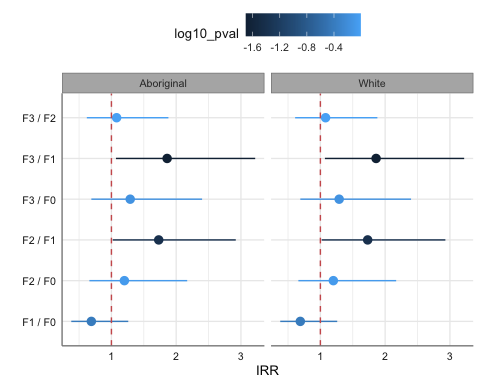# 5 Survival Analysis We will use an example on the effect of thiotepa versus placebo on the development of bladder cancer. data(bladder) bladder <- bladder %>% mutate(times = stop, rx = factor(rx, labels=c("Placebo", "Thiotepa")) ) %>% var_labels(times = "Survival time", rx = "Treatment") ## 5.1 Parametric method model_surv <- survreg(Surv(times, event) ~ rx, data = bladder) Using robust standard errors: model_surv %>% glm_coef(labels = c("Treatment: Thiotepa/Placebo", "Scale"), se_rob = TRUE) %>% as_hux() %>% set_align(everywhere, 2:3, "right") %>% theme_pubh(1) %>% add_footnote(get_r2(model_surv), font_size = 9) ParameterSurvival time ratioPr(>|z|) Treatment: Thiotepa/Placebo1.64 (0.89, 3.04)0.116 Scale1 (0.85, 1.18)0.992 Nagelkerke's R2 = 0.02 In this example the scale parameter is not statistically different from one, meaning hazard is constant and thus, we can use the exponential distribution: model_exp <- survreg(Surv(times, event) ~ rx, data = bladder, dist = "exponential") model_exp %>% glm_coef(labels = c("Treatment: Thiotepa/Placebo"), se_rob = TRUE) %>% as_hux() %>% set_align(everywhere, 2:3, "right") %>% theme_pubh(1) %>% add_footnote(get_r2(model_exp), font_size = 9) ParameterSurvival time ratioPr(>|z|) Treatment: Thiotepa/Placebo1.64 (0.85, 3.16)0.139 Nagelkerke's R2 = 0.02 Interpretation: Patients receiving Thiotepa live on average 64% more than those in the Placebo group. Using naive standard errors: model_exp %>% glm_coef(labels = c("Treatment: Thiotepa/Placebo")) %>% as_hux() %>% set_align(everywhere, 2:3, "right") %>% theme_pubh(1) %>% add_footnote(get_r2(model_exp), font_size = 9) ParameterSurvival time ratioPr(>|z|) Treatment: Thiotepa/Placebo1.64 (1.11, 2.41)0.012 Nagelkerke's R2 = 0.02 model_exp %>% plot_model(type = "pred", terms = ~ rx, dot.size = 1.5, title = "") %>% gf_labs(y = "Survival time", x = "Treatment", title = "")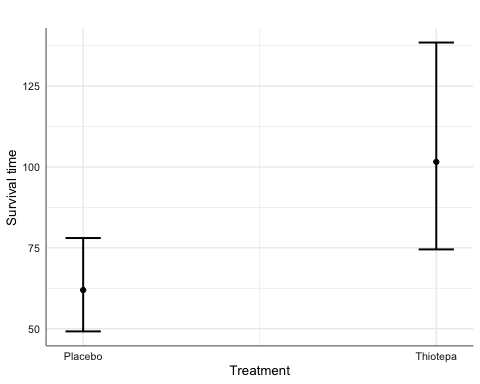## 5.2 Cox proportional hazards regression model_cox <- coxph(Surv(times, event) ~ rx, data = bladder) model_cox %>% glm_coef(labels = c("Treatment: Thiotepa/Placebo")) %>% as_hux() %>% set_align(everywhere, 2:3, "right") %>% theme_pubh(1) %>% add_footnote(get_r2(model_cox), font_size = 9) ParameterHazard ratioPr(>|z|) Treatment: Thiotepa/Placebo0.64 (0.44, 0.94) 0.02 Nagelkerke's R2 = 0.016 Interpretation: Patients receiving Thiotepa are 64% less likely of dying than those in the Placebo group. model_cox %>% plot_model(type = "pred", terms = ~ rx, dot.size = 1.5, title = "") %>% gf_labs(x = "Treatment", title = "")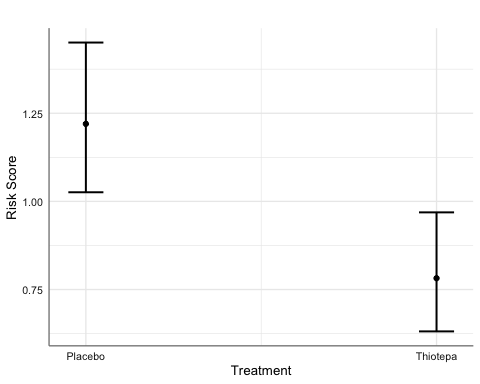# 6 Mixed Linear Regression Models ## 6.1 Continuous outcomes We look at the relationship between sex and age on the distance from the pituitary to the pterygomaxillary fissure (mm). require(lme4, quietly = TRUE) data(Orthodont, package = "nlme") Orthodont <- Orthodont %>% var_labels( distance = "Pituitary distance (mm)", age = "Age (years)" ) We fit the model: model_mix <- lmer(distance ~ Sex * age + (1|Subject), data = Orthodont) model_mix %>% summ(center = TRUE, confint = TRUE, model.info = FALSE)  AIC 445.76 BIC 461.85 Pseudo-R² (fixed effects) 0.41 Pseudo-R² (total) 0.78 Fixed Effects Est. 2.5% 97.5% t val. d.f. p (Intercept) 24.97 24.02 25.92 51.38 25.00 0.00 Sex -2.32 -3.81 -0.83 -3.05 25.00 0.01 age 0.78 0.63 0.94 10.12 79.00 0.00 Sex:age -0.30 -0.54 -0.07 -2.51 79.00 0.01 p values calculated using Satterthwaite d.f. ; Continuous predictors are mean-centered. Random Effects Group Parameter Std. Dev. Subject (Intercept) 1.82 Residual 1.39 Grouping Variables Group # groups ICC Subject 27 0.63 model_mix %>% center_mod() %>% glm_coef(labels = c( model_labels(model_mix), "Sex:Age interaction" )) %>% as_hux() %>% set_align(everywhere, 2:3, "right") %>% theme_pubh(1) %>% add_footnote(get_r2(model_mix), font_size = 9) ParameterCoefficientPr(>|t|) Constant24.97 (24.02, 25.92)< 0.001 Sex: Female-2.32 (-3.81, -0.83)0.005 Age (years)0.78 (0.63, 0.94)< 0.001 Sex:Age interaction-0.3 (-0.54, -0.07)0.014 Conditional R2 = 0.783 Marginal R2 = 0.41 Effect plot: model_mix %>% plot_model("eff", terms = age ~ Sex, show.data = TRUE, jitter = 0.1, dot.size = 1.5) %>% gf_labs(y = get_label(Orthodontdistance), x = "Age (years)", title = "")